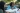# Mastering the Three Trigonometric Ratios: Sin Cos Tan

POSTED ON MARCH 10, 2023The three trigonometric ratios, sin cos tan, lay down the foundations for trigonometry. Through these ratios, you can evaluate trigonometric expressions, find unknown measures, and solve problems involving right triangles. This article will be your guide to mastering what sin cos tan represents.

## What Does the Trigonometric Ratios Sin Cos Tan Represent?

Sin cos tan represents the three most important ratios in trigonometry: sine, cosine, and tangent. These three ratios are based on the ratios formed by two sides of a right triangle. They are extremely useful when it comes to finding unknown sides and angles of a right triangle.

Before getting excited, why don’t we begin with the basics? Start by understanding the key components of the ratios and identifying the specific lengths involved with finding the value of sine, cosine, and tangent of a given angle.Juni Learning has excellent resources that will help you in mastering trigonometry concepts. Discover the different techniques and methods offered by Juni Learning’s content to support you in mastering Common Core math topics and concepts. Juni Learning will not only help you in math, but it can be your overall ally when it comes to learning core concepts for other subjects!

In a right triangle and with a reference angle, say A, there are three crucial components:

• Opposite side: the side facing opposite to angle A.

• Hypotenuse: the longest side of any right triangle.

This means that through sin cos tan, we can now relate one angle of a triangle with its two sides.Boost math confidence to the next level
Juni’s vetted instructors study at top US Universities and provide our students with the support and mentorship to grow their math skills.

Let’s break down the three ratios: sine, cosine, and tangent.

• The sine of an angle, say θ, is equal to the ratio of the side opposite θ over the right triangle’s hypotenuse. A great way to remember “SOH”, where S for Sine, O for Opposite, and H for Hyptenuse.• Similarly, the cosine of θ is equal to the ratio of the side adjacent to θ over the right triangle’s hypotenuse. Now, easily remember this relationship using “CAH”: C for Cosine, A for Adjacent, and H for Hypotenuse.• Tangent of θ is equal to the ratio of the side opposite θ over the side adjacent to θ. Like the two other ratios, remember the relationship using “TOA”: T for Tangent, O for Opposite, and A for Adjacent.This table summarizes the relationships between sin cos tan - plus a reminder to use the guide: SOH CAH TOA!Our advisors can answer any questions you have about our curriculum or subscriptions. They can even provide a course recommendation.

#### Example 1

What are the sin cos tan values of A for the right triangle shown below?Identify the important sides of the right triangle when finding the values of sin A, cos A, and tan A.

1. The side opposite angle A has a length of 8 cm.

2. The adjacent side relative to A has a length of 6 cm.

3. The hypotenuse (longest side of the right triangle) is 10 cm long.

Now, apply the relationships established for sin cos tan and evaluate the values that we need.This shows that through the relationships between the angles and sides of a right triangle, it is now possible to evaluate the values of sin cos tan. In fact, for this problem, we have A= 4/5, A= 3/5, and A= 4/3.

## Using Sin Cos Tan To Find the Exact Values

Recall that we have two special right triangles in Trigonometry: the 45-45-90 and 30-60-90 triangles. These triangles’ sides have special ratios of 1:1:√2 and 1:√3:2, respectively. By using the rules for sin cos tan, it’s now possible to find the exact trigonometric values for the following angles: 30°, 45°, and 60°.#### Example 2

Find the exact values of the following expressions:

a. sin 30°

Use the 30-60-90 triangle since you’re looking for the exact value of 30°. Identify the side facing opposite 30° and the length of the hypotenuse.Simplify the ratio to find the exact value of 30°. This shows that 30°= 1/2.

b. cos 45°

Apply a similar approach to evaluate 45°, but this time, use the 45-45-90 triangle.The side adjacent to 45° is 1 unit long while the hypotenuse is √2 unit long. Simplifying this leads to 45°= 1/√2.

## Final Thoughts

Juni Learning has excellent resources for exploring the new landscape of math learning and comprehension. If you’d like to encourage more interaction with lessons in Common Core’s mathematical standards, visit Juni Learning to explore their options. Just like those math standards, Juni Learning’s catalog can encourage exploration in other subjects, too!

Juni Learning is also launching new tutoring help. The Juni Tutor Bot is an AI-powered tutoring solution designed to provide personalized help to students for homework help and test preparation. The bot is available on Discord, a popular communication platform, and can be accessed by signing up for the Juni Tutor Bot. Once signed up, students can chat with the Juni Tutor Bot and receive customized help based on their unique learning needs.